#Quality Digest

Featured Product
This Week in Quality Digest Live
Statistics Features
Donald J. Wheeler
What do predictable processes have in common with chaos?
William A. Levinson
People can draw the wrong conclusions due to survivor, survey, and bad news bias.
Donald J. Wheeler
Does your approach do what you need?
Paul Laughlin
Correlation vs. causality
Donald J. Wheeler
In spite of what everyone says to the contrary
Statistics News
New capability delivers deeper productivity insights to help manufacturers meet labor challenges
Day and a half workshop to learn, retain, and transfer GD&T knowledge across an organization
Elsmar Cove is a leading forum for quality and standards compliance
InfinityQS’ quality solutions have helped cold food and beverage manufacturers around the world optimize quality and safety
User friendly graphical user interface makes the R-based statistical engine easily accessible to anyone
Collect measurements, visual defect information, simple Go/No-Go situations from any online device
Good quality is adding an average of 11 percent to organizations’ revenue growth
Ability to subscribe with single-user minimum, floating license, and no long-term commitment
A guide for practitioners and managersBio

Statistics

## Short Run SPC, Part 1

### Tracking the process while making different products

Published: Monday, December 2, 2019 - 13:03

All articles in this series:

Lean production is built on the explicit assumption that each step is operated predictably and capably. Predictable operation can only be achieved and maintained by using process behavior charts. But short production runs and multiple products make it hard to see how to use process behavior charts in a lean environment. So what can be done? This paper will show how to use a zed chart for individual values to track your process while it is making different products.

### Unit 12

Unit 12 is used to make Products 1201 and 1202. The final characteristic for Product 1201 has a target value of 19.0, while the final characteristic for Product 1202 has a target value of 8.0. Batches 43 through 72 contain short runs of both products.Figure 1: Data for Unit 12

Of course a naive approach would be to simply place the data from Unit 12 on an XmR chart as shown in figure 2.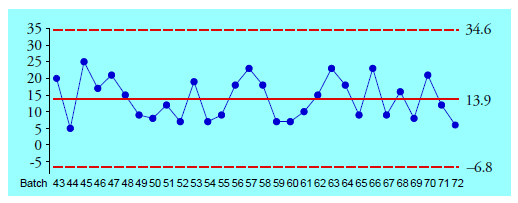Figure 2: Incorrect X chart for Batches 43 through 72

Of course the problem with figure 2 is that it combines apples and oranges. The concept of rational subgrouping that applies to all types of process behavior charts requires the successive values of an XmR chart to be logically comparable. Here we are deliberately changing between Product 1201 and Product 1202 at various times. The different target values guarantee that successive values will not all be logically comparable, and the structure of the chart in figure 2 is irrational.

Could we compute separate limits for each product? Yes, but if we leave the data in time-order we end up with the chart in figure 3.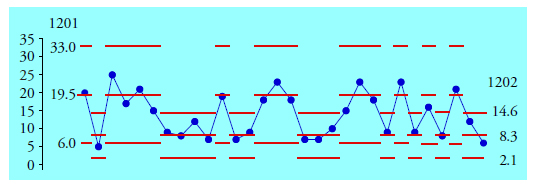Figure 3: X chart for Unit 12 with limits for each product

While this chart is correct point-by-point, it is a visual mess. And this chart only tracks two products. One plant manager told me he made 30 products in two reactors. Just imagine what his version of figure 3 would have looked like! So, we need a different approach to the problem of tracking the process while making different products.

### Short run charts

Process behavior charts may be easily adapted to short production runs. The two main requirements for making these adaptations are an appreciation of the fact that reasonable limits may be obtained from small amounts of data, and an understanding of how to use the charts to discover new information rather than merely confirming that which is already known.

The two types of short run charts for individual values are zed charts and difference charts. This paper will illustrate the zed chart which is a completely general approach to the problem of operating a process predictably while making different products. The difference chart, which only works under certain conditions, will be the subject of Part Two of this series.

Since Unit 12 produces two products we will begin by summarizing the product specific information by creating baseline XmR charts for each product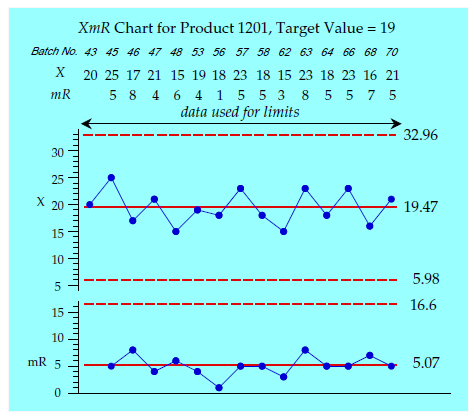Figure 4: Baseline XmR chart for Product 1201

Batches 43 through 70 for Product 1201 have an average of 19.47 units, and an average moving range of 5.07 units. Dividing by d2 = 1.128 standard deviations we obtain Sigma(X) = 4.495 units per standard deviation for Product 1201. Thus it takes about 4.5 measurement units to equal one standard unit of dispersion for Product 1201.Figure 5: Baseline XmR chart for Product 1202

Batches 44 through 72 for Product 1202 have an average of 8.33 units, and an average moving range of 2.36 units. Dividing by d2 = 1.128 standard deviations we obtain Sigma(X) = 2.092 units per standard deviation for Product 1202. Thus it takes about 2.1 measurement units to equal one standard unit of dispersion for Product 1202.

How many data are needed for these baselines? When operating with short production runs it may take some time to accumulate data for some products. The product specific charts above have baselines of 15 values each. When possible, baselines of 15 to 20 values for each product specific chart are preferred. However, when necessary, limits and estimates may be computed using as few as 5 or 6 values per product. When using these short baselines the limits and estimates may be revised as additional values are obtained for each product. Once your limits and estimates are based on 20 or more values there will be little need for further revision.

### Zed charts

The zed chart makes allowances for different aim points and different amounts of variation from product to product. In order to use a zed chart we will have to have both a nominal value and a Sigma(X) value for each product.

The nominal values may be either target values or baseline average values. Since the idea of short runs presumes that production can switch back and forth between products, I prefer to use the target values for the nominal values. If production is doing a reasonable job of operating on target the product averages should generally be found within one-half Sigma(X) of the target values. Here the baseline averages of 19.47 and 8.33 are close to the target values of 19.0 and 8.0.

As is always the case with process behavior charts we absolutely, positively must use within-subgroup measures of dispersion. When working with individual values this means using two-point moving ranges as illustrated above. For the construction of a zed chart all other methods of computing measures of dispersion are incorrect.

A zed chart uses zed-scores in place of the original data. An original value for a given product will be transformed into a zed-score by first subtracting off that product’s nominal value, and then by dividing this difference by that product’s Sigma(X) value.

To allow for verification that the transformations are done correctly it is good practice always to record the original raw values on the zed chart. In addition, a table or record of the nominal values and Sigma(X) values for each product should be included with a zed chart.

For Product 1201, with the target value of 19 as our nominal value, and with Sigma(X) = 4.49, the zed transform will be: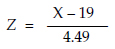For Product 1202, with the target value of 8 as our nominal value, and with Sigma(X) = 2.09, the zed transform will be:These zed transforms convert the deviations from the nominal value from measurement units into standard deviation units. They express each value as some number of standard deviation units above or below the nominal. Having converted the original data into zed-scores, we can then place the zed scores for apples, oranges, and bananas side by side on a zed chart.

The central line for the zed chart will be 0.00, and the limits will be placed at ±3.00. A point that falls outside these limits will indicate an item that is detectably different from the nominal value, and it will be different enough to be worth the trouble to find out why.

The moving ranges are computed using the zed scores. Traditionally this (standardized) range value has been denoted by the symbol W. These moving range values are plotted on a W-chart having a central line of 1.128 and an upper limit of:The zed chart for Unit 12 (Batches 73 through 92) is shown in figure 6.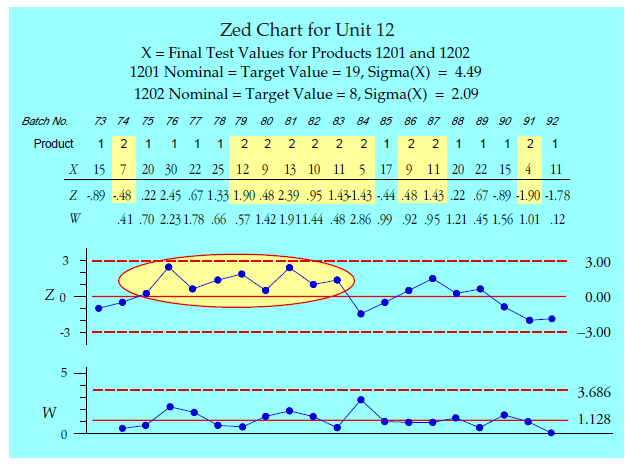Figure 6: Zed chart for Unit 12

On the zed chart in figure 6 we notice a long run above the central line beginning with Batch 75. This long run is not apparent on the updated product specific charts in figures 7 and 8.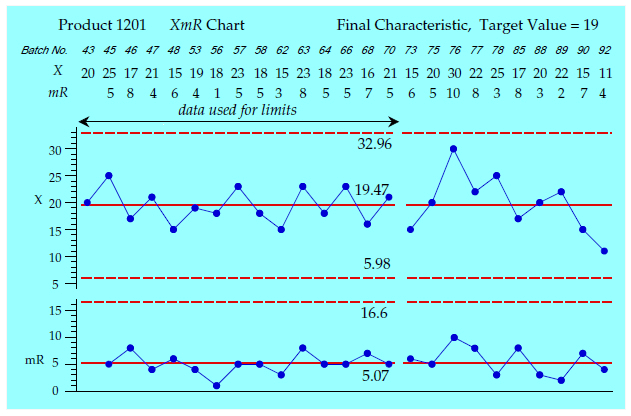Figure 7: XmR chart for Product 1201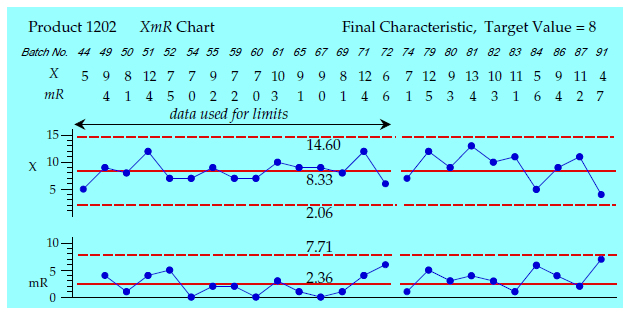Figure 8: XmR chart for Product 1202

So, between Batch 75 and Batch 84 there was some special cause present which affected the aim of the process. If we use this knowledge to discover the assignable cause, we will have discovered something about how to better operate this process. If we ignore this signal on the zed chart, then we will have missed an opportunity to have learned something about this process.

### A zed chart done wrong

As a second example we use a zed chart from a plant in Europe. Three products are produced in one unit, and each product has a different target value. Figure 9 shows the standardized chart produced at the plant. The different colors represent the different products.Figure 9: “Standardized” chart for 65 batches of three products

No points fall outside the limits, but those who are accustomed to looking at process behavior charts will immediately recognize that the running record does not look right. The empirical rule tells us to expect anywhere from 60 percent to 75 percent of our data within one sigma of the central line. The highlighted one-sigma zone above contains 53 out of 65 batches, which is 82 percent!

To illustrate this problem another way figure 10 shows the histograms for the values in figure 9. All three histograms display the same problem, but it is most clearly seen in the histogram for Product Green.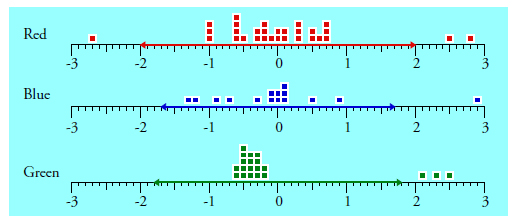Figure 10: Histograms of values from figure 9

The 20 batches for Product Green are spread out just over 3 sigma, which is reasonable for a histogram of 20 values with one long tail. But the lower 17 batches are all squeezed into less than one-half sigma. This is a problem because we expect a histogram of 17 values to be spread out between 3 and 4 sigma since the average range for 17 values is d2 = 3.588 sigma.

In a similar manner the lower 14 batches of Product Blue have a range of just over 2 sigma rather than the expected d2 = 3.407 sigma. And the middle 27 batches of Product Red have a range of under 2 sigma rather than the expected d2 = 4.027 sigma.

What causes this squeezing of the middle of the histograms? It is the result of using an inflated measure of dispersion in the standardization of these data.

Classes in statistics generally teach that we standardize the values in a data set by subtracting the average and then dividing by the global standard deviation statistic. However, this “standardization transformation” has a prerequisite that the data must be independent and identically distributed. This little assumption that precedes so much of what we do in statistics means that the standardization transformation is only appropriate when the data are homogeneous.

Whenever you use a global standard deviation statistic you are making a very strong assumption that the data are completely homogeneous. When those data are not homogeneous the global standard deviation statistic will be inflated, and when you divide by this inflated number the middle portion of your histogram will be squeezed as shown in figure 10.

How can you avoid this problem? When creating a zed chart for individual values your estimate of dispersion must always be based on either an average two-point moving range or a median two-point moving range.

### A zed chart done right

Fortunately, the plant also provided the original measurements along with the incorrect zed chart of figure 9.Figure 11: Data from plant in Europe

Using these measurements we get the product specific XmR charts of figure 12.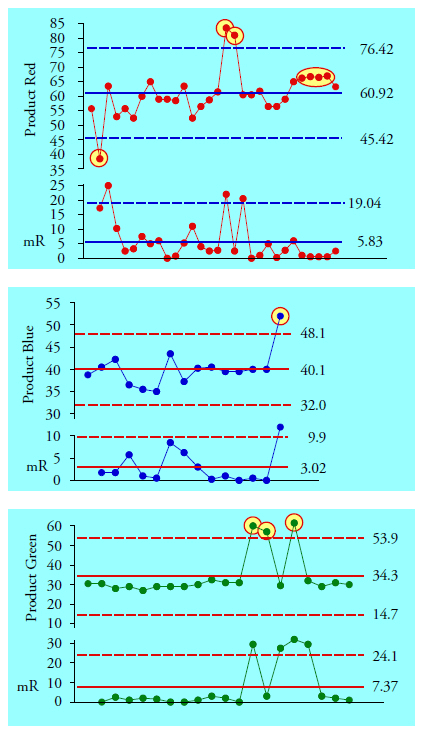Figure 12: XmR charts for Products Red, Blue, and Green

The three X-charts in figure 12 contain seven out-of-limits points and one run beyond one-sigma. Why don’t we see these signals in figure 9? The over-standardization created by using the global standard deviation statistics effectively buried all of these signals.

The use of within-subgroup measures of dispersion to filter out the noise within the data is perhaps the greatest statistical breakthrough of the 20th century. It is the foundation for all modern techniques of statistical analysis.  And it is a foundation that we cannot afford to ignore when creating a zed chart. When working with individual values, we must use two-point moving ranges as our basis for computing limits to detect signals within the data.

Moreover, since the idea of producing different products in succession using the same equipment requires production personnel to change the process aim, it makes sense to use the target values rather than the baseline averages when computing the zed values. Thus, the zed transformations for the three products are:

Product Red has a target value of 60 and an average moving range of 5.828, which gives a Sigma(X) value of 5.83/1.128 = 5.167. (Rather than the s = 8.17 used when producing figure 9.)

Product Blue has a target value of 40 and an average moving range of 3.016, which gives a Sigma(X) value of 3.016/1.128 = 2.674. (Rather than the s = 4.06 used when producing figure 9.)

Product Green has a target value of 30 and an average moving range of 7.367, which gives a Sigma(X) value of 7.367/1.128 = 6.531. (Rather than the s = 10.95 used when producing figure 9.)

When we compute the zed values using the target values and Sigma(X) values given above we get the correct zed chart in figure 13.Figure 13: Zed chart for 65 batches of three products

Here we find all of the signals seen in figure 12 plus an additional run below one sigma. About half of these batches (33/65) are pretty much right on target. About one-fourth of these batches (16/65) show evidence of the process being operated detectably off-target. These off-target batches involve all three products and are evidence of assignable causes of exceptional variation that prevent the process from making the desired products. So the problems do not appear to be related to any single product. But could these problems be due to the product change-overs?Figure 14: Moving range chart for the zed chart

The moving ranges for the zed scores are shown on the mW chart in figure 14. There the black dots represent the ranges for the changes between products, while the colored dots represent the within-product ranges. Of the eleven ranges that fall above the upper limit, only the four that  are circled come from the change-overs between products. So the problems here do not appear to be unique to the product change-overs. The exceptional values may occur at any time, with any product.

Thus, we have to conclude the problems here are fundamental to the production process itself, regardless of the product being produced.

### Standardization vs. zed

The traditional standardization transformation taught in introductory classes in statistics consists of subtracting the average value and dividing by the global standard deviation statistic. While this transformation is appropriate for data that are independent and identically distributed (i.e., homogeneous) it does not work satisfactorily with data that are not homogeneous. When the data are not homogeneous they will generally contain signals. Here the standardization transformation will squeeze the middle portion of the histogram to accommodate the extreme values. This will tend to bury the signals, and may also affect the average value, as happens above with Product Green.

The zed transformation is completely different from the traditional standardization transformation. The nominal value subtracted from the data is usually a target value. But the estimate of dispersion in the denominator must be some within-subgroup dispersion statistic. As illustrated here the difference is considerable. Do not confuse the two.

While the zed transformation will allow you to detect signals contained within the data, you should not expect it to produce a bell-shaped histogram out of a collection of nonhomogeneous data. The idea here is to identify the signals, not produce pretty histograms.

### Summary

A zed chart allows us to place apples, oranges, and bananas together on a single chart so that we can visualize the underlying process even when it is being used to make different products.

We adjust for differences in aim by subtracting the target value for each product from the data for that product. We adjust for differences in variation by dividing by a within-subgroup measure of dispersion for each product. So while a zed chart may sound more complex than an ordinary process behavior chart, it is fairly simple in practice. In between obtaining a raw value and plotting a point, you merely have to subtract the appropriate, product specific target value and divide by the appropriate, product specific Sigma(X) value.

The target values are generally part of the specifications, and we find the within-subgroup measures of dispersion from the product specific baseline charts.

Since the zed transformation is not the same as the traditional standardization transformation taught in introductory classes in statistics, be sure your software uses the zed transformation rather than the standardization transformation when creating a zed chart.

### Discuss